# F_un linear algebra

Posted by on Sep 22, 2008 in graduate, KapranovSmirnovNo comments

This text was posted on June 10th, 2008 at neverendingbooks

Today we will define some basic linear algebra over the absolute fields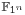following the Kapranov-Smirnov document. Recall from last time thatand that a d-dimensional vectorspace over this field is a pointed setwhereis a free-set consisting of n.d elements. Note that in absolute linear algebra we are not allowed to have addition of vectors and have to define everything in terms of scalar multiplication (or if you want, the-action). In the hope of keeping you awake, we will include an F-un interpretation of the power residue symbol.

Direct sums of vectorspaces are defined via, that is, correspond to the disjoint union of free-sets. Consequently we have that.

For tensor-product we start withthe vectorspace cooresponding to the Cartesian product of free-sets. If the dimensions ofandare respectively d and e, thenconsists of n.d.n.e elements, so is of dimension n.d.e. In order to have a sensible notion of tensor-products we have to eliminate the n-factor. We do this by identifying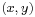withand call the corresponding vectorspace. If we denote the image ofbythen the identification merely says we can pull the-action through the tensor-sign, as we’d like to do. With this definition we do indeed have that.

Recall that any linear automorphismof anvectorspacewith basis(representants of the different-orbits) is of the formfor some powers of the primitive n-th root of unityand some permutation. We define the determinant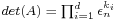. One verifies that the determinant is multiplicative and independent of the choice of basis.

For example, scalar-multiplication bygives an automorphism on any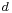-dimensional-vectorspaceand the corresponding determinant clearly equals. That is, the det-functor remembers the dimension modulo n. These mod-n features are a recurrent theme in absolute linear algebra. Another example, which will become relevant when we come to reciprocity laws :

Take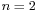. Then, avectorspaceof dimension d is a set consisting of 2d elementsequipped with a free involution. Any linear automorphismis represented by a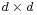matrix having one nonzero entry in every row and column being equal to +1 or -1. Hence, the determinant.
On the other hand, by definition, the linear automorphismdetermines a permutation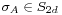on the 2d non-zero elements of. The connection between these two interpretations is that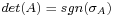the determinant gives the sign of the permutation!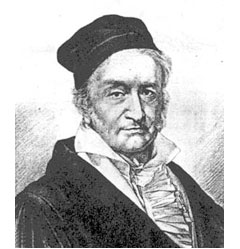For a prime powerwith, we have seen that the roots of unityand hence thatis a vectorspace over. For any field-unit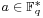we have the power residue symbol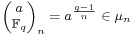On the other hand, multiplication byis a linear automorphism on the-vectorspaceand hence we can look at its F-un determinant. The F-un interpretation of a classical lemma by Gauss asserts that the power residue symbol equals.

An-subspaceof a vectorspaceis a subset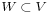consisting of full-orbits. Normally, in defining a quotient space we would say that two V-vectors are equivalent when their difference belongs to W and take equivalence classes. However, in absolute linear algebra we are not allowed to take linear combinations of vectors…

The only way out is to defineto correspond to the free-setobtained by identifying all elements of W with the zero-element in. But… this will screw-up things if we want to interpret-vectorspaces as-spaces whenever.

For this reason, Kapranov and Smirnov invent the notion of an equivalencebetween-spaces to be a linear map (note that this means a set-theoretic mapsuch that the invers image of 0 consists of full-orbits and is a-map elsewhere) satisfying the properties thatand for every elementwe have that the number of pre-imagesis congruent to 1 modulo n. Observe that under an equivalencewe have that.

This then allows us to define an exact sequence of-vectorspaces to be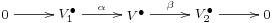witha set-theoretic inclusion, the composition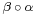to be the zero-map and with the additional assumption that the map induced by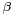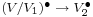is an equivalence. For an exact sequence of spaces as above we have the congruence relation on their dimensions.

More importantly, if as beforeand we use the embeddingto turn usual-vectorspaces into absolute-spaces, then an ordinary exact sequence of-vectorspaces remains exact in the above definition.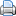Print This Post